# Maths Grey Cells at the ready: Feynman and Newton Did not Point This Out....What am I missing?

If anyone can find a flaw in this - i’d be grateful…

I was looking at Richard Feynman’s Lecture - The Relationship of Mathematics to Physics Equal areas in equal times where he ‘borrows’ from Newton’s Principia an argument about showing equal areas are swept out in equal times, it is all very entertaining… but I could not help but think “WHY SO COMPLICATED?”

Why did Feynman not ‘just’ SAY and show that a right-angle triangle of height equal to an arc length of a circle is the same area as the area of the arc length fraction of the circle. Did Newton not know two pi radius equals Circumference? I think he did. Or that Pi times radius squared was equal to the area?

I don’t get it.

Then if the particle / planet / whatever is going at the same speed it will sweep out the same area from a fixed point whether it is going straight or being bent by gravity or at the end of a string from a circle.

So maybe I am wrong… but I cooked up a few images for my own use using my roman room …idea

If anyone can find a flaw in this - i’d be grateful.
It is not a proof of course, Pi wasn’t called Pi in Newton’s day but Feynman knew…

Maybe this is not common knowledge??

Thanks for looking and using your grey cells.

2 Likes

Um… Not watched it, but is it something simple, like the fact that a perfectly circular orbit is unlikely for a planet, and that when it’s closer to the gravity source, it moves faster, sweeping out a greater arc at a closer proximity, which means it accounts for the same area per unit time, so the triangle relationship isn’t so useful, because you’re not exactly describing circles, but the area thing helps describe speed in some way, or something like that?

So, really, the area kinda helps describes the speed or acceleration, and it’s more mathematically manipulable and intuitive as an arc description than as an ever changing triangle, maybe?

1 Like

That’s my first reaction too - you seem to be assuming a couple of things that aren’t true:

1. Planets move in circles
2. Planets move at a constant speed
1 Like

Thanks for the input, couple of things though: I am not assuming anything. This works for an ellipse as well. (area of ellipse from memory is Pi times half the lengths of both axis.). And, perhaps more to the point - Feynman’s Lecture is specifically about a circular orbit. I probably don;t have to remind anyone here (as we have all memorised it) that a circle is just a special case of an ellipse where the two axis are equal.

Why do you say Feynman’s lecture is specifically about a circular orbit? He shows that if the force is directed towards the sun, then equal areas will be swept out in equal time. There are no assumptions about the shape of the orbit.

Hi Simon, thanks for your interest and reply, much appreciated. I think we are talking at cross purposes a little here, as you are asking me questions about why… I say this and that.

However, since you ask and seem dubious (I do hope that does not cause you any offence) the reason I say Feynman lecture is specifically about a circle as it is specifically in the lecture in the transcript and also in the book of the lectures on this topic.

He talks of “If a planet is going in a circle…” (Direct quote from the lecture)…

Here’s a screenshot of the book: where he is discussing (as he does in the video) swinging a stone around the head on a string - which is exactly what I was trying to get across in this post.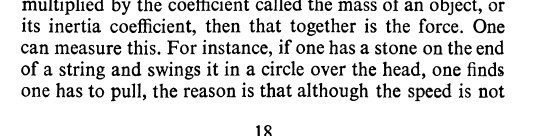All of this of course is linked to deriving the way in which calculus is taught - Newton did not use calculus in his proof, (though he did use it to do the work I am told - then he made geometrical proofs to justify his calculus work), my point is it would have been a lot simpler to tell people about equal areas and right angled triangles to get the idea across instead of the long winded geometrical proof which needs knowledge of parallel lines and tangents…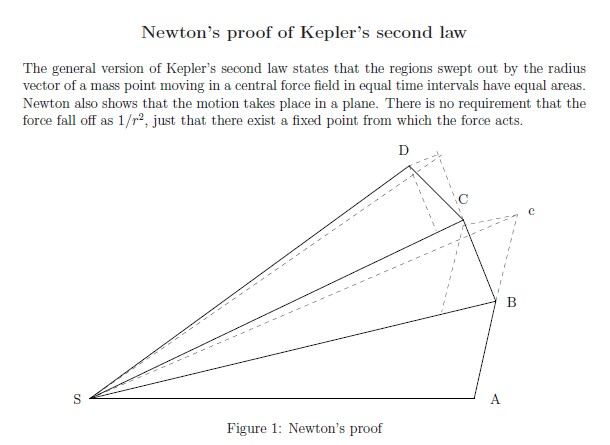The Calculus proof is this: (well one of them) I can think of much MUCH simpler ways of doing this as well. I am primarily interested in how to introduce people to new ideas - and in that I think simplicity and what people are familiar with from school might is a good way to proceed.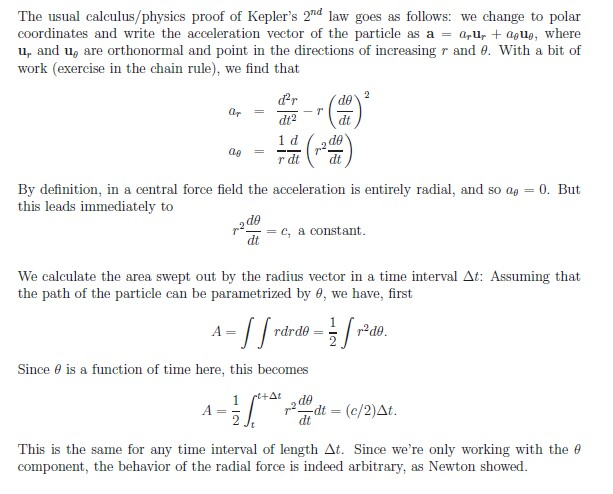That’s a nice proof as it has the variable for acceleration for radial motion shown as zero - and leads to a constant - which is exactly the same as saying the length of the arc is the same as the height of the triangle and results in a constant of 1:1 proportion.

K

1 Like

Hi K,

Thanks for the detailed explanation! I still disagree, thoughThe mentions of a planet going in a circle and swinging a stone in a circle are just simple examples to explain that a centripetal force can produce a curved orbit. Those examples aren’t even in the same lecture as the equal areas demonstration, so I’m not sure why you would think the idea of a circular orbit extends to that.

As I said, there are no assumptions about the shape of the orbit in Newton/Feynman’s argument. If the force is directed towards the sun, equal areas are swept out in equal time. The orbit doesn’t have to be circular or elliptical. It could be a weird wiggly shape - as long as the force is always directed towards the sun, equal areas will be swept out in equal time.

Hi Simon,
I think it best to leave this here, your …

That doesn’t wash.

You claim it is not the same lecture, oh really?

The fact is it is the same lecture. As the lecture, the transcript and the book bear testimony to, so you are fooling yourself, but not me or anyone else.

This was a post about clarification to make a proof easier to understand for people taking up calculus. I take it you are not ‘into’ calculus? That’s fine, but my explanation making it simpler to introduce is - well it’s junior maths.

Arc length straightened as the height of a right angled triangle with base the same as the radius - take the area and it is the same (exactly) as the percentage of the arc of the area of a circle (or an ellipse).

The maths is simple but your disagree (stamping your feet) but you have not provided any maths to support your objection. If the force is directed towards the sun is exactly the same as saying that the second derivative of the position d2s/dt^2 = zero and therefore the corresponding integral (the area swept out) is equal in equal times. If you don;t know what d2s/dt^2 means, then … learn it.

That’s not true. The shape of the ORBIT is proved by the maths (above the calculus part).
It also holds good for a circle - the one that Feynman was talking about.

I think that because I worked it out (see above). The equation is x^2+y^2=radius. = 1

Incidentally, I am a bit intrigued by this:

No two body problem can be a weird wiggly shape. Unless you know different.

I do apologise if this seems harsh, but… there is nothing worse than someone fooling themselves, and I get this impression from your post.

As for memory systems - tell kids (and anyone else) this fact about areas before they are intoruced to integrals and differentials - it will help.

Thanks again.

k

Hi K,

Thanks for the reply. No offence taken. It seems like we are both arguing in good faith, and we strongly disagree, so this is kind of fun!

I have a first class honours degree in Physics - not to appeal to authority, but just to let you know that I’m not a novice in this area.

About it being a different lecture - Feynman gave a series of lectures on The Character of Physical Law. The mention of circles happens in the first lecture in the series, and the equal areas demonstration is in the second lecture a week later.

As for the orbit shape - in the real world, the force is proportional to the square root of the distance, which does produce elliptical orbits. However, consider a case where the force changes in a different way - maybe even randomly over time. The orbit could be any arbitrary wiggly shape, and the equal areas law would still hold, as long as the force was always directed towards the sun.

To clarify, I don’t disagree with any of your maths, just the relevance of your maths to what Newton and Feynman are demonstrating: that if a force is directed towards a point, equal areas are swept out in equal time. This is true regardless of the size of the force or the shape of the orbit, and their proof holds regardless of the size of the force or the shape of the orbit.

1 Like

Sorry to hear you can’t see the relevance of the maths to what newton and Feynman are saying.

It is the same maths.

Pretty weird Physics course you did if you can’t follow the maths and also think that an orbit can go in a weird wiggly shape. Where exactly did you get this First Class Degree in Physics? Off eBAY? Hogwarts? Imaginaryland?

And don’t worry, you don’t come across to me as any sort of authority.

K

Newton’s argument shows that area is swept out at a constant rate by a moving object under a force directed towards a fixed point.

This result holds regardless of the nature or magnitude of the force. As stated in the note above the diagram you pasted, “there is no requirement that the force fall off as 1 / r^2, just that there exist a fixed point from which the force acts”. The force could be proportional to 1 / r, or 1 / r^3, or even \sin(t) where t is the time since I last had breakfast - as long as the force is always directed towards (or away from) the fixed point, equal areas will be swept out in equal time.

When it comes to gravity, of course, the force is proportional to 1 / r^2, and the orbit is an ellipse. But the equal areas in equal time result applies for any centrally directed force.

To summarise, the Newton/Feynman geometrical argument and the calculus argument you posted both show that a centrally directed force implies equal areas swept in equal time.

Your geometrical argument (as presented in the first post) shows the same thing, but only in the case where the object is moving at constant speed in a circular orbit.

1 Like

This is nonsense.

Really Simon, I am sorry to be blunt, but you really should not fool yourself into thinking this make sense.

You are comparing Apples with Orangutangs.

From what you have written, it seems you do not have a clue what you are talking about. I think you THINK you know and want others to THINK that you know, but anyone with your claimed qualification - what was it again?

I would have thought, would have hoped actually that anyone who has such a qualification should know (without thinking) that your statement:

Is immediately and obviously wrong.

Why is it immediately obvious?

Because: There is no ‘TIME’ in my diagram (or in Newton’s geometrical Proof!) - it is only about areas (units squared). I mean where do you get the idea about ‘constant speed’ from???

The area swept out has nothing to do with the speed it was swept out has it?

If you don’t believe me and can’t work it out yourself (like what I did…- on paper) here’s a quote from that proof above. Note the comment about “THE RESULT WOULD STILL HOLD”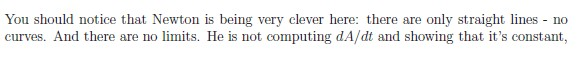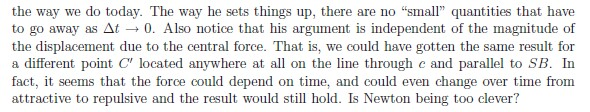Similarly, this approach I outlined above - also works for an ellipse or any curved shape which you have a formula for… While it is true I used the equation of a circle x^2 + y^2 = r^2, (here r=1 which I then used for the base of the relative triangle) I could have used an ellipse equally as well. (x^2/a^2)+(y^2/b^2)=1. Where the area will be accurate to less than 0.2 % - (that’s 99.81596479% accurate to be exact) the reason for the 0.02% error I don’t have to explain as you will know it from the work you did on integrals during your Physics course…

K

That quote about how the force could change over time and Newton’s argument would still hold is exactly what I’ve been saying! Do you agree that a force that varies over time could produce a wiggly orbit, in which equal areas would still be swept out in equal time?

Back to your diagram - I got the idea of constant speed from the fact you have a circular arc of 30 degrees and are matching the curved distance with the straight-line distance. Surely that would only be valid if the speed didn’t change along the curved path?

Prove it then. On paper. And post it here. I’ve given enough free maths lessons to you.

I’ve now muted you I am afraid First Class Honours Boy.

Bye!

I’m sorry we didn’t come to a resolution here. For whatever reason, it seems like we aren’t communicating well.

If I may make a suggestion, perhaps you could ask your original question on https://physics.stackexchange.com.